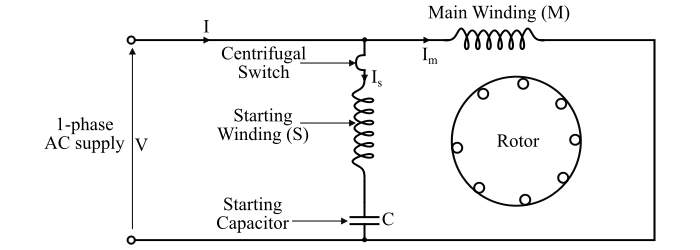# Capacitor-Start Induction Motor ŌĆō Characteristics and Applications

A capacitor-start induction motor is a type of single-phase induction motor in which the starting torque is produced by using a starting capacitor and an auxiliary winding. The capacitor-start induction motor consists of a starting winding (S) and a main or running winding (M). Both the winding have the same number of turns. A starting capacitor (C) is connected in series with the starting winding as shown in the circuit diagram of the motor.The value of the starting capacitor is so chosen that the starting current Is leads the main winding current Im by an angle (α) of 90°. The starting torque of the capacitor-start induction motor is given by,

$$\mathrm{τ_{­ØæĀ­ØæĪ} = ­Øæś­ØÉ╝_{­ØæĀ}­ØÉ╝_{­ØæÜ}\:sin\:\alpha = ­Øæś­ØÉ╝_{­ØæĀ}­ØÉ╝_{­ØæÜ}}$$

As the value of phase angle (α) is very high (α = 90°), consequently, the capacitor-start induction motor has very high starting torque.As soon as the speed of the motor reaches about 75% of the synchronous speed, then the centrifugal switch isolates the starting winding from the motor circuit. Now, the motor operates as a 1-phase induction motor and continues to accelerate till it reaches the normal speed. The normal speed of the motor depends upon the load on the motor.

## Characteristics of Capacitor-start Induction Motor

Following are the primary characteristics of a capacitor-start induction motor −

• The capacitor-start induction motor develops a very high starting torque about 3 to 4.5 times of the full-load torque.

• The value of the starting capacitor must be large and the starting winding resistance low to obtain a starting torque.

• The capacitor-start induction motor requires the starting capacitor of high VAR rating, thus, the electrostatic capacitors of the order of 250 F are used.

• The starting capacitor is a short-time rated capacitor.

• The capacitor-start induction motors possess good starting and running characteristics.

• For a capacitor-start induction motor, the starting current is small and the starting torque is high. Therefore, starting winding of a capacitor-start induction motor heats up less quickly and is well suited to the application where the starting period is either small or prolonged.

## Applications of Capacitor-Start Induction Motor

The capacitor-start induction motors are used to drive the loads of high inertia where frequent starts are required such as −

• For driving pumps and compressors

• Used in refrigerators and in air-conditioner compressors.

• Used for conveyors and machines tools, etc.